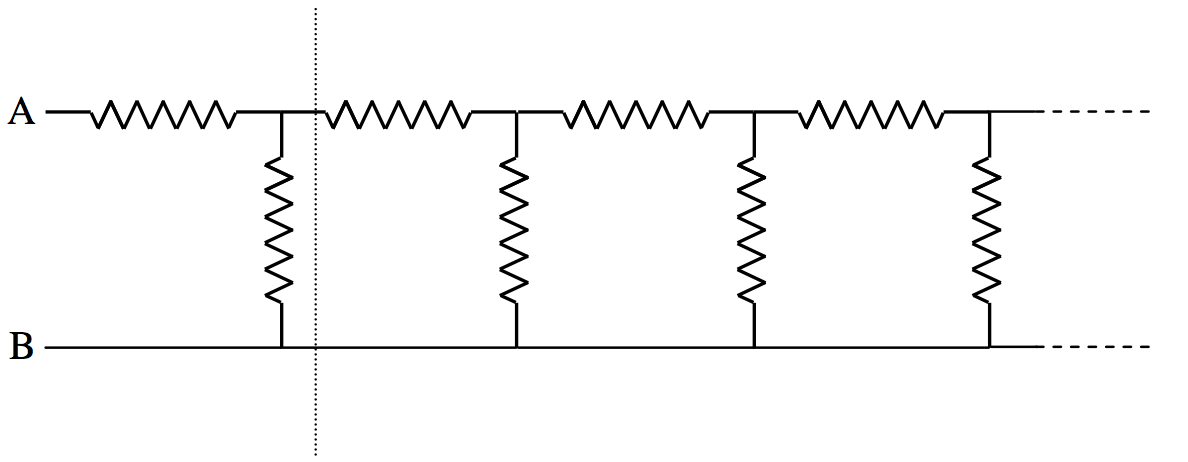$$\require{cancel}$$

# 4.14: Tortures for the Brain

•• Contributed by Jeremy Tatum
• Emeritus Professor (Physics & Astronomy) at University of Victoria

I don’t know if any of the examples in this section have any practical applications, but they are excellent ways for torturing students, or for whiling away rainy Sunday afternoons.

# Q4.14.1The drawing shows 12 resistances, each of value r $$\Omega$$, arranged along the edges of a cube. What is the resistance across opposite corners of the cube?

# Q4.14.2The drawing shows six resistors, each of resistance 1 $$\Omega$$, arranged along the edges of a tetrahedron. A 12 V battery is connected across one of the resistors. Calculate the current between points A and B.

# Q4.14.3The figure shows six resistors, whose resistances in ohms are marked, arranged along the edges of a tetrahedron. Calculate the net resistance between C and D.

# Q4.14.4

R1 = 8 $$\Omega$$ and R2 = 0.5 $$\Omega$$ are connected across a battery. The rate at which heat is generated is the same whether they are connected in series or in parallel. What is the internal resistance r of the battery?

# Q4.14.5

R1 = 0.25 $$\Omega$$ and R2 = ? are connected across a battery whose internal resistance r is 0.5 $$\Omega$$. The rate at which heat is generated is the same whether they are connected in series or in parallel. What is the value of R2?

# Q4.14.6In the above circuit, each resistance is 1 ohm. What is the net resistance between A and B if the chain is of infinite length?

# Q4.14.7

What is the resistance between A and B in question 4.14.6 if the chain is not of infinite length, but has n “links” – i.e. 2n resistors in all?

# Q4.14.8

In the circuit below, what is the potential difference between A and B, and what is the current in each resistor?